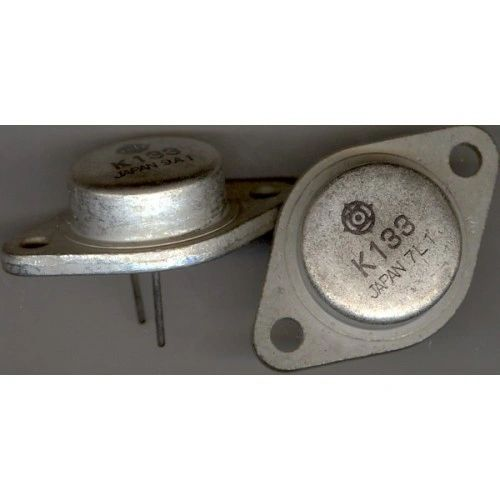•# TRANSISTOR K133 NPN-Output dc-Input Optocoupler Part Number = K133P

\$38.00 \$27.00
Free Shipping!

TRANSISTOR

K133

NPN-Output dc-Input Optocoupler

Part Number = K133P
Manufacturer Name = Vishay Telefunken
Description = NPN-Output dc-Input Optocoupler
Number of Channels = 1
V(BR)CEO (V) Breakdown Voltage = 32
I(C) Abs.(A) Collector Current = 100m
P(D) Max.(W) Power Dissipation = 150m
V(CE)sat Max.(V) = 0.4
@I(C) (A) (Test Condition) = 2.0m
@I(F) (A) (Test Condition) = 20m
I(F) Max. (A) Forward Current = 60m
V(F) Max.(V) Forward Voltage = 1.3
@I(F) (A) (Test Condition) = 20m
CTR Min.(%)Current Trans.Ratio = 25
@I(F) (A) (Test Condition) = 10m
t(resp) Max.(s) Response Time = 20u
Viso Max.(V) Isolation Voltage = 10k
Package = TO-116
Military = N

THIS SALE IS FOR 1 EACH

........

NOTE:

ALL ELECTRONICS ARE UNTESTED

SOLD AS IS